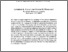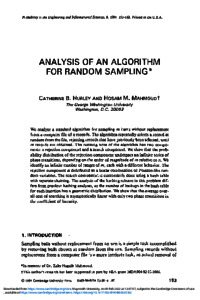# Analysis of an Algorithm for Random Sampling

Hurley, Catherine B. and Mahmoud, Hosam M. (1994) Analysis of an Algorithm for Random Sampling. Probability in the Engineering and Informational Sciences, 8. pp. 153-168. ISSN 0269-9648Previewmore...Add this article to your Mendeley library

## Abstract

We analyze a standard algorithm for sampling m items without replacement from a computer file of n records. The algorithm repeatedly selects a record at random from the file, rejecting records that have previously been selected, until m records are obtained. The running time of the algorithm has two components: a rejection component and a search component. We show that the probability distribution of the rejection component undergoes an infinite series of phase transitions, depending on the order of magnitude of m relative to n. We identify an infinite number of ranges of m, each with a different behavior. The rejection component is distributed as a linear combination of Poisson-like random variables. The search component is customarily done using a hash table with separate chaining. The analysis of the hashing scheme in this problem differs from previous hashing analyses, as the number of lookups in the hash table for each insertion has a geometric distribution. We show that the average overall cost of searching is asymptotically linear with only two phase transitions in the coefficient of linearity.

Item Type: Article analysis; algorithm; random sampling; Faculty of Science and Engineering > Mathematics and StatisticsFaculty of Science and Engineering > Research Institutes > Hamilton Institute 15466 Dr. Catherine Hurley 09 Feb 2022 12:11 Probability in the Engineering and Informational Sciences Cambridge University Press YesItem control page